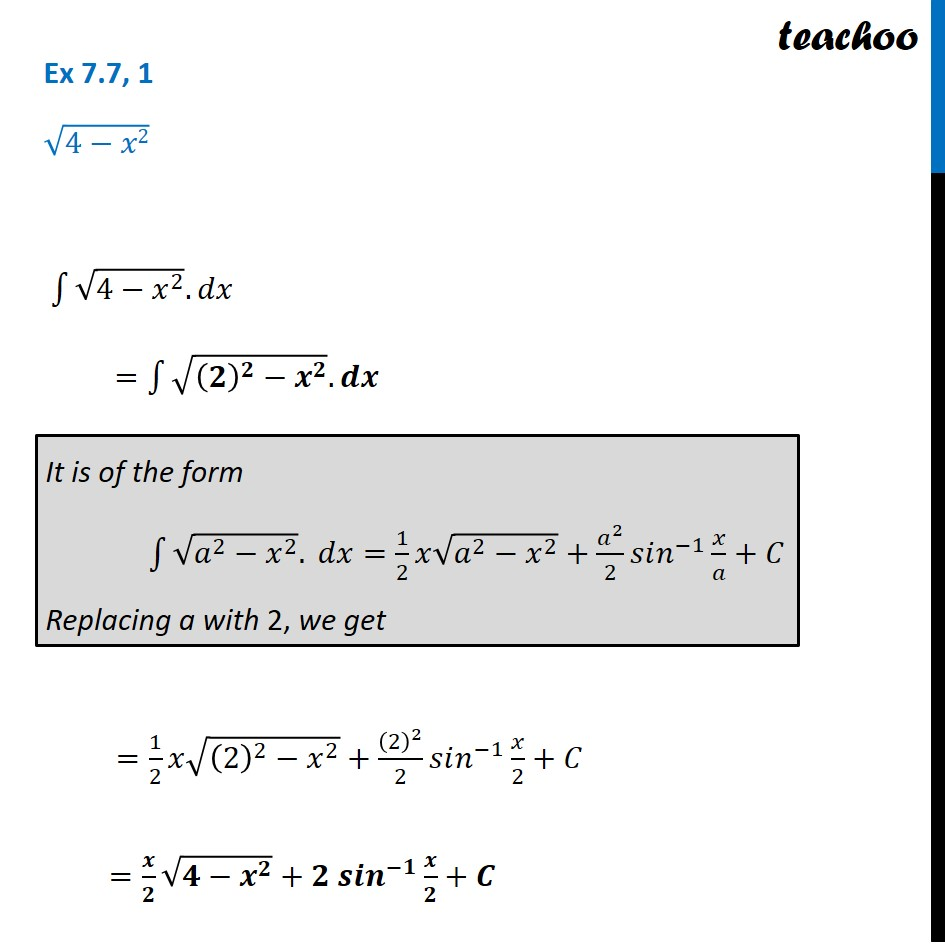Ex 7.7

Chapter 7 Class 12 Integrals
Serial order wiseLearn in your speed, with individual attention - Teachoo Maths 1-on-1 Class

### Transcript

Ex 7.7, 1 √(4−𝑥2) ∫1▒〖√(4−𝑥^2 ).𝑑𝑥〗 =∫1▒〖√((𝟐)^𝟐−𝒙^𝟐 ).𝒅𝒙〗 =1/2 𝑥√((2)^2−𝑥^2 )+(2)^2/2 𝑠𝑖𝑛^(−1) 𝑥/2+𝐶 =𝒙/𝟐 √(𝟒−𝒙^𝟐 )+𝟐 𝒔𝒊𝒏^(−𝟏) 𝒙/𝟐+𝑪 It is of the form ∫1▒〖√(𝑎^2−𝑥^2 ). 𝑑𝑥=1/2 𝑥√(𝑎^2−𝑥^2 )+𝑎^2/2 𝑠𝑖𝑛^(−1) 𝑥/𝑎+𝐶〗 Replacing a with 2, we get